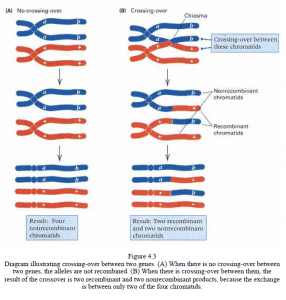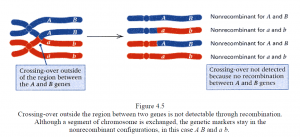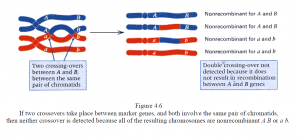# Genetic Mapping

Genetic Mapping

• The linkage of the genes in a chromosome can be represented in the form of a genetic map, which shows the linear order of the genes along the chromosome with the distances between adjacent genes proportional to the frequency of recombination between them.
• A genetic map is also called a linkage map or a chromosome map. The concept of genetic mapping was first developed by Morgan’s student, Alfred H. Sturtevant, in 1913.
• The early geneticists understood that recombination between genes takes place by an exchange of segments between homologous chromosomes in the process now called crossing-over.
• Each crossing-over is manifested physically as a chiasma, or cross-shaped configuration, between homologous chromosomes; chiasmata are observed in prophase I of meiosis.
• Each chiasma results from the breaking and rejoining of chromatids during synapsis, with the result that there is an exchange of corresponding segments between them.
• The theory of crossing-over is that each chiasma results in a new association of genetic marker. When there is no crossing-over, the alleles present in each homologous chromosome remain in the same combination.
• When crossing-over does take place, the outermost alleles in two of the chromatids are interchanged (recombined). The unit of distance in a genetic map is called a map unit; 1 map unit is equal to 1 percent recombination.
• For example, two genes that recombine with a frequency of 3.5 percent are said to be located 3.5 map units apart.
• One map unit is also called a centimorgan, abbreviated cM, in honor of T. H. Morgan. A distance of 3.5 map units, therefore, equals 3.5 centimorgans and indicates 3.5 percent recombination between the genes.
• The four completely equivalent ways in which a genetic distance between two genes may be represented.
1. As a frequency of recombination (in the foregoing example, 0.035)
2. As a percent recombination (here 3.5 percent)
3. As a map distance in map units (in this case, 3.5 map units)
4. As a map distance in centimorgans (here 3.5 centimorgans, abbreviated 3.5 cM)• Physically, 1 map unit corresponds to a length of the chromosome in which, on the average, one crossover is formed in every 50 cells undergoing meiosis.
• If one meiotic cell in 50 has a crossing-over, the frequency of crossing-over equals 1/50, or 2 percent.
• Yet the frequency of recombination between the genes is 1 percent. The correspondence of 1 percent recombination with 2 percent crossing-over is a little confusing until you consider that a crossover results in two recombinant chromatids and two nonrecombinant chromatids.
• A frequency of crossing-over of 2 percent means that of the 200 chromosomes that result from meiosis in 50 cells, exactly 2 chromosomes (the two involved in the exchange) are recombinant for genetic markers spanning the particular segment.• To put the matter in another way, 2 percent crossing-over corresponds to 1 percent recombination because only half the chromatids in each cell with an exchange are actually recombinant.
• In situations in which there are genetic markers along the chromosome, such as the A, a and B, b pairs of alleles, recombination between the marker genes takes place only when crossing-over occurs between the genes, rather than between the genes A and B.
• The crossing-over does result in the physical exchange of segments between the innermost chromatids. However, because it is located outside the region between A and B, all of the resulting gametes must carry either the A B or a b allele combinations. These are non-recombinant chromosomes.
• The presence of the crossing-over is undetected because it is not in the region between the genetic markers. In some cases, the region between genetic markers is large enough that two (or even more) crossovers can be formed in a single meiotic cell.• In this example, both crossovers are between the same pair of chromatids. The result is that there is a physical exchange of a segment of chromosomes between the marker genes, but the double crossover remains undetected because the markers themselves are not recombined.
• The absence of recombination results from the fact that the second crossover reverses the effect of the first, insofar as recombination between A and B is concerned. The resulting chromosomes are either A B or a b,] both of which are nonrecombinant.
• Given that double crossing-over in a region between two genes can remain undetected because it does not result in recombinant chromosomes, there is an important distinction between the distance between two genes as measured by the recombination frequency and as measured in map units.
• Map units measure how much crossing-over takes place between the genes. For any two genes, the map distance between them equals one-half times the average number of crossover events that take place in the region per meiotic cell.
• The recombination frequency, on the other hand, reflects how much recombination is actually observed in a particular experiment. Double crossovers that do not yield recombinant gametes, do contribute to the map distance but do not contribute to the recombination frequency.
• The distinction is important only when the region in question is large enough that double-crossing over can occur. If the region between the genes is so short that no more than one crossover can be formed in the region in any one meiosis, then map units and recombination frequencies are the same (because there are no multiple crossovers that can undo each other).
• This is the basis for defining a map unit as being equal to 1 percent recombination. Over an interval so short as to yield 1 percent observed recombination, multiple crossovers are usually precluded, so the map distance equals the recombination frequency in this case.References: